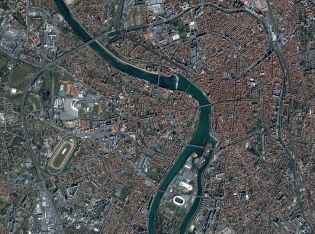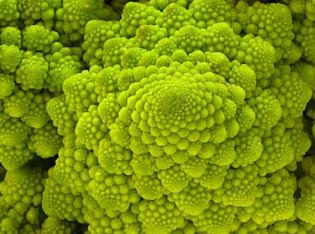# Fractals

## The Von Koch SnowflakeAutomatic translation Category: matter and particles
Updated June 01, 2013

If we replace the infinite, the middle third of each side of a triangle with two equal length segments, we obtain a fractal figure. Whatever you are doing zoom image we observe the same details. The characteristic of a fractal is that the perimeter tends to infinity, adds detail since increasingly smaller as and when successive iterations. Yet this curve does not overlap any time limits of a circle which circumscribes the initial triangle. Fractal geometry has allowed us to understand that nature obeys a simple mathematical law. Since one can understand this concept, translating what we see in nature in mathematical language.

### Image: variant of classical fractal curve of Von Koch published in 1904, usually called « snowflake Koch ».## Fractal object

Fractal and fractal object are terms from the Latin adjective "fractus" which means "irregular or broken". The word was created in 1975 by Benoit Mandelbrot in the first edition of his book.
The concept itself begins in the 50s and has a fascinating side because it leads to some form of infinity.
In 1967, Mandelbrot published in the journal 'Science' a famous article "How long is the coast of Britain?".
He describes a new object of fractional dimension.
If a straight line is an object of dimension 1 and a 2 dimensional plane, the Brittany coast has a dimension of 1, xx or xx describes the roughness.
It is the same for the bark of trees.
The intestinal wall has a dimension greater than 2 and less than 3, as the bronchi of the lungs or the branching of blood vessels.
As Von Koch snowflake, the length of the coast of Britain is infinite.
In the Von Koch curve details are exactly the same whatever the scale, because if you look at a part of this figure it is impossible to say, if one looks at scale 1, or if we made a zoom from 10 to 100 or infinite.
In nature the details of a fractal object are similar but not identical. The concept of infinity makes fascinating fractals, to the point that the creators of fractal images were widely exploited this concept by using the power of computers. The result proves unpredictable because it is directly related to initial conditions. The slightest inaccuracy, however small, to the extent of initial parameters is amplified to such an extent that the state reached by the system after a certain time, can be completely chaotic.

Mandelbrot has created a new field for describing the structure of objects and natural phenomena or man-made.

### Image: The Mandelbrot set. Benoit Mandelbrot is a Franco-American mathematician, born in Warsaw, November 20, 1924 and died October 14, 2010 in Cambridge, Massachusetts. He developed a new class of mathematical objects: fractals, or fractal.## Fractal Geometry of Nature### Image: The spider structure of galaxy clusters is a representation of a fractal object.### Image: The structure of our cities is a representation of a fractal model.### Image: The Romanesco broccoli is a beautiful natural representation of a fractal model.

 1997 © Astronoo.com − Astronomy, Astrophysics, Evolution and Earth science. Contact    Mentions légalesDoes the black mater exist?Are we alone in the universe?non-baryonic dark matter...